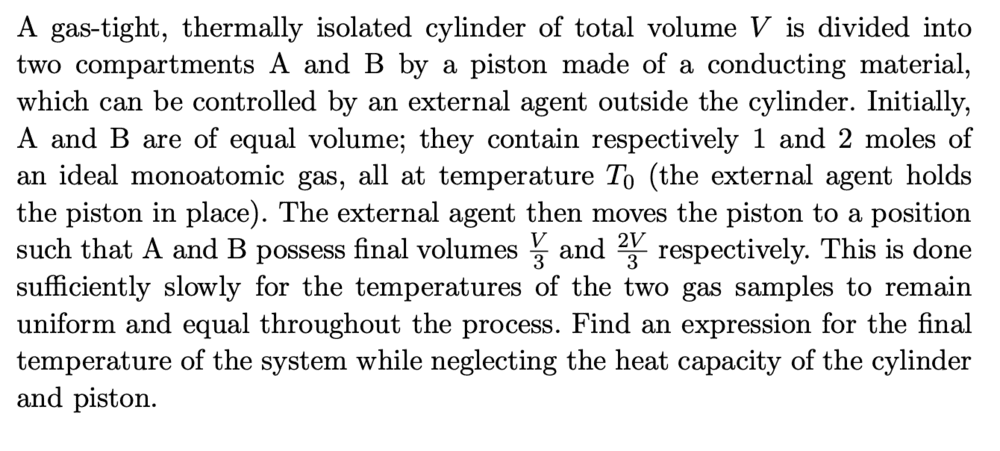# When do formulas for adiabatic processes apply?

• phantomvommand

#### phantomvommand

Homework Statement
Relevant Equations
Change in internal energy = nCvTIn this problem, the method used to solve the question is to equate pdV with change in internal energy. This implies an adiabatic process as Q = 0? (not sure about this claim) However, why is it not correct to simply apply the PV^ϒ = constant formula?

Thank you.

why is it not correct to simply apply the PV^ϒ = constant formula?
What would you apply that to? You cannot apply it to the system as a whole since there are two initial pressures. You cannot apply it to one partition since there is heat flow between them.

•phantomvommand